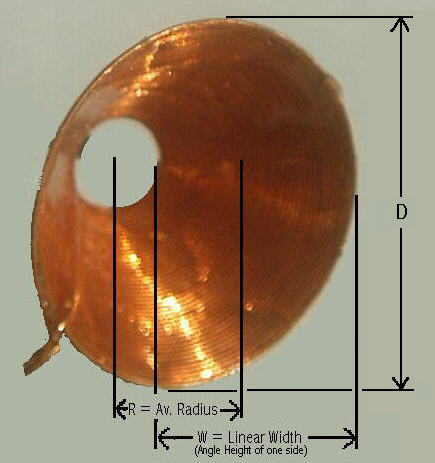# My New Conical Coil Inductance Calculator

For Calculation of inductance of Conical type coils, simular to this one shown here
Here is My Formula: (((N * R) * (N * R)) / ((8 * R) + (11 * W)))-DNNumberofTurns RAvg.Radius(inches) WLinearWidth of Coil(inches) DOutsideDiameter(inches)
 LCalculatedInductance(uH)